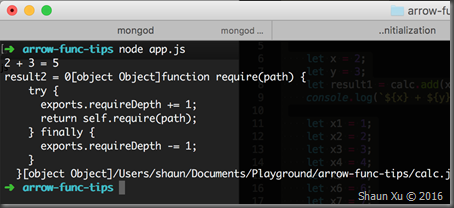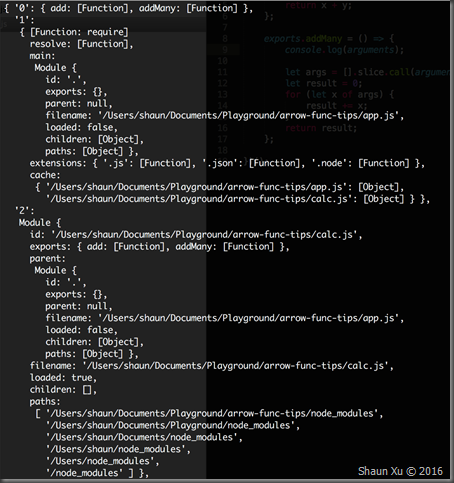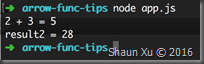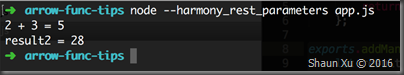# Shaun Xu

## The Sheep-Pen of the Shaun

### NewsShaun, the author of this blog is a semi-geek, clumsy developer, passionate speaker and incapable architect with about 10 years’ experience in .NET and JavaScript. He hopes to prove that software development is art rather than manufacturing. He's into cloud computing platform and technologies (Windows Azure, Amazon and Aliyun) and right now, Shaun is being attracted by JavaScript (Angular.js and Node.js) and he likes it.

Shaun is working at Worktile Inc. as the chief architect for overall design and develop worktile, a web-based collaboration and task management tool, and lesschat, a real-time communication aggregation tool.• Posts - 122

## Pay Attention to Use ES6 Arrow Function with 'arguments'

There is an enhancement in ECMAScript 6 named "Arrow Functions", which likes lambda expression in C#, allow us to define a function within less lines of code. I like this new feature and began to use it in my Node.js application as many as I could. But today when II  was using JavaScript build-in "arguments" variant I found something wrong.

Assuming we have a module to add parameters, which is very simple. I'm using Arrow Functions to implement as below.

```// calc.js

(() => {
'use strict';

exports.add = (x, y) => {
return x + y;
};
})();```

Then I can use it as below.

```// app.js

(() => {
'use strict';

const calc = require('./calc.js');

let x = 2;
let y = 3;
console.log(`\${x} + \${y} = \${result1}`);

})();```

Now I created another method in my module allows user to input multiple numbers to add. In traditional JavaScript way I don't need to define arguments in the function. I can use "arguments" variant, which is a "semi-array" object contains parameters, add each of them and return the summary.

```// calc.js
(() => {
'use strict';

exports.add = (x, y) => {
return x + y;
};

let args = [].slice.call(arguments);
let result = 0;
for (let x of args) {
result += x;
}
return result;
};

})();

// app.js
(() => {
'use strict';

const calc = require('./calc.js');

let x = 2;
let y = 3;
console.log(`\${x} + \${y} = \${result1}`);

let x1 = 1;
let x2 = 2;
let x3 = 3;
let x4 = 4;
let x5 = 5;
let x6 = 6;
let x7 = 7;
let result2 = calc.addMany(x1, x2, x3, x4, x5, x6, x7);
console.log(`result2 = \${result2}`);

})();```

But when I ran this application I got an error below.

I'm using Node.js v5.7.0 which supports ES6 features.If we read Arrow Function specification carefully we will find that it captures the "this" value of the enclosing context, so the following code works as expected. This provides convenient to use parent "this" inside arrow function without needing to specify another variant to hold parent's "this" value. But the side effect is, it also captures the "arguments" value from the parent context.

In my code I defined "addMany" function in arrow function mode. It copied "this" from parent context, which is the whole module, as well as "arguments", which is the module loading function arguments.To fix this problem, just simply define this function normally as below. It will use its own "this" and "arguments".

```exports.addMany = function () {
let args = [].slice.call(arguments);
let result = 0;
for (let x of args) {
result += x;
}
return result;
};```Alternatively, if you are OK to enable one of Node.js ES6 staging features called "Rest Parameters" you can define the function as below, which allows parameters to be passed in as a real array.

```exports.addMany = (...args) => {
let result = 0;
for (let x of args) {
result += x;
}
return result;
};```

Then execute this application with Node.js options called "--harmony_rest_parameters".Hope this helps,

Shaun

All documents and related graphics, codes are provided "AS IS" without warranty of any kind.# re: Pay Attention to Use ES6 Arrow Function with 'arguments' Posted by sobhana123 on 5/28/2018 1:58 PM hi. I don,t now please tell me any one thanks for sharing this topic.ISO 9001 in Oman